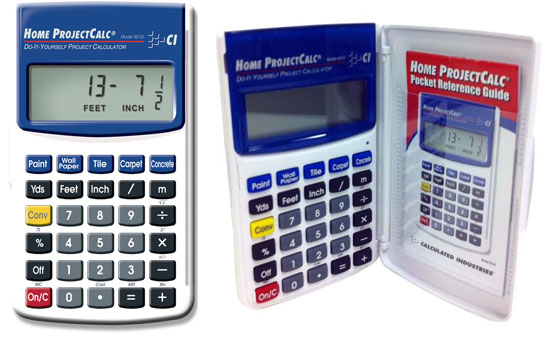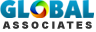# Home ProjectCalc for house construction

## Home » Construction take-off sheets » Earthwork Volume Calculation Sheet

Presenting you the home Project Calculator, which normally helps to design, apply, install and finish the home development projects. It will grow the confidence of the home cost estimator.

You can get what you exactly need. It can instantly calculate the paint and wallpaper covering.

The tiles and concrete measurement can also be calculated.

The money and the materials which are the most important part of any construction can be measured here.

It will save the time and considerably decrease calculation error, calculation errors and make fewer trips to the home improvement store.

How it helps:-

• It can calculate material costs for paint, wallpaper, concrete along tile
• The measurement is in feet, inches, fractions, decimal fractions, yards as well as meters.
• Convert all standard to math dimensions.
• Standard math is : +, -, +/-, x, ÷, %, p, X2, and v. - all included in calculator.

Enter measurements just as you say it in feet, inches, fractions, decimal fractions, yards and meters. Convert between all standard math dimensions.# Takeoff Tools